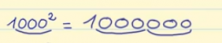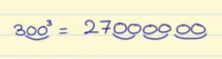Smartick is an advanced online program that teaches kids math and coding in only 15 min. a day

Feb22

# Powers of Base 10, Base 100 and Numbers Followed by Zeros

## Powers

In this post, we are going to learn how to raise numbers to powers when the base is composed of zeros. Remember that powers serve to allow us to write a multiplication problem of the same number several times in a simpler way.

For example, 4x4x4x4x4.

We are multiplying the number 4 a total of 5 times. To put this multiplication in the form of powers we first write the 4 and then above and to the right we write a small number 5.

4 5

### Powers of base 10

The exponent indicates how many zeros we should put after the result.

Examples:

10 2 → Since the exponent is 2 we have to put 2 zeros: 10 2 = 100

10 5 → Since the exponent is 5 we have to put 5 zeros: 10 5 = 100,000

### Powers of base 100

Since 100 has 2 zeros we have to write two zeros as many times as the exponent indicates.

Examples:

100 2→ Since the exponent is 2 we have to write 2 zeros twice:

1 00 00

100 2  = 10,000

100 4 → Since the exponent is 4 we have to write 2 zeros four times:

1 00 00 00 00

100 4  = 100,000,000

For any base with more zeros we should carry out the same procedure: take note of the number of zeros in the base and repeat that number of zeros as many times as the number that there is in the exponent.### Powers of numbers followed by zeros

If the base is a number followed by zeros we only have to raise the number to the exponent and then put as many zeros as we need.

Examples:

20 3  → First we calculate 23 = 8 then we add 3 zeros:

20 3  = 8,000If you have enjoyed this post and want to learn more check out Powers in Math

And if you want to learn more math, register for a Smartick free trial today!